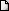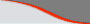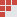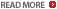IIM CAT Math questions and answers. CAT, XAT Quant Questions in Inequalities : Ascent - CAT Classes, MockCATsLATEST NEWS • Ascent Success Stories • TANCET 16 Classes @ Chennai • XAT 16 Classes @ ChennaiHome | TANCET Coaching Class | TANCET study material | Online TANCET Course | Contact us
 Ascent's Courses TANCET Coaching Chennai Online TANCET Course TANCET MBA Study Material CAT Classroom Chennai CAT Correspondence India Mock CAT Series GRE Coaching Chennai GMAT Coaching Chennai Online GMAT CourseUS B Schools Faculty List Jobs @ Ascent Testimonials Ascent Success Stories Contact Us +91 44 4500 8484 +91 96000 48484
You are here: Home » CAT Questions » Inequalities » Domains and Ranges

#### Inequalities : Algebra - CAT 2016 Preparation

Question 4 the day : May 16, 2006

The question for the day is a sample practice problem in Inequalities, an Algebra Topic. The problem provides an understanding of the concept of finding out the range of values of "x" that satisfy a given inequality.

Question
Which of the following inequalities have a finite range of values of "x" satisfying them?
1. x2 + 5x + 6 > 0
2. |x + 2| > 4
3. 9x - 7 < 3x + 14
4. x2 - 4x + 3 < 0
Correct Answer - x2 - 4x + 3 < 0. Choice (4)

We have to find out the values of "x" that will satisfy the four inequalities given in the answer choices and check out the choice in which the range of values satisfying is finite.

Choice 1
Factorizing the given equation, we get (x + 2)(x + 3) > 0.
This inequality will hold good when both x + 2 and x + 3 are simultaneously positive or simultaneously negative.

Evaluating both the options, we get the range of values of "x" that satisfy this inequality to be x < -2 or x > -3. i.e., "x" does not lie between -2 and -3 or an infinite range of values.

Choice 2
|x + 2| > 4 is a modulus function and therefore, has two options
Option 1: x + 2 > 4 or
Option 2: (x + 2) < -4.
Evaluating the two options we get the values of "x" satisfying the inequality as x > 2 and x < -6. i.e., "x" does not lie between -6 and 2 or an infinite range of values.

Choice 3
9x - 7 < 3x + 14
Simplifying, we get 6x < 21 or x < 3.5. Again an infinite range of values.

Choice 4
x^2 - 4x + 3 < 0
Factorizing we get, (x - 3)(x - 1) < 0.

This inequality will hold good when one of the terms (x - 3) and (x - 1) is positive and the other is negative.
Evaluating both the options, we get 1 < x < 3. i.e., a finite range of values for "x".

Hence, choice 4 is the correct answer.

## XLRI XAT TANCET Practice Question Samples

TANCET Questionbank
 TANCET MBA MCA Practice Questions with detailed explanation and shortcuts.GMAT Math Books
 Comprehensive study material for GMAT Quant. 1800+ questions.TANCET Diagnostic Test

 CAT Online Course

 GMAT Diagnostic Test

 GMAT Math Lesson Book | SAT prep | GRE Classes @ Chennai | GMAT Coaching @ Chennai | TANCET Mailing List © 2002 - 17 ASCENT Education all rights reserved. Terms & Condition | Privacy Policy